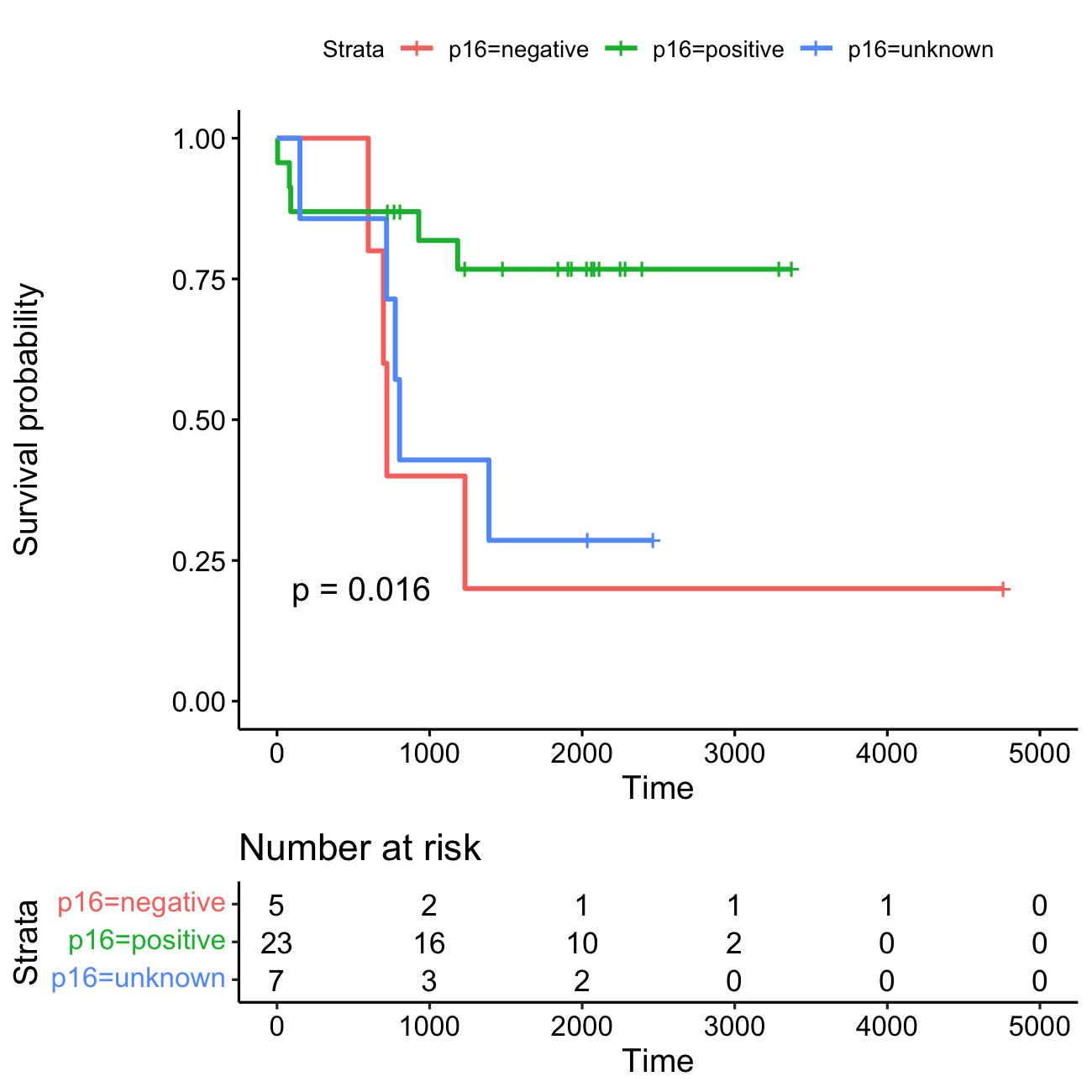# Kaplan-Meier Curve using R

Published:

Kaplan-Meier curve shows what the probability of an event (for example, survival) at a certain time interval. The log-rank test compares the survival curves of two or more groups. With a small subset of patients, the Kaplan-Meier estimates can be misleading and should be interpreted with caution.

KM Analysis using R The packages used for the analysis are survival and survminer. Use install.packages( ) to install these libraries just in case if they are not pre installed in your R workspace.

library(survival)
library(survminer)
library(dplyr)


base.dir = "/Users/adinasa/Documents/Nabil/survival_analysis"


Examine the dataset (Vital status: 1 for dead; 0 for alive)

head(data)

ID      p16 		Status	Days
GHN-11  unknown		1  	803
GHN-15  unknown		1  	775
GHN-20  unknown		1  	150
GHN-21  unknown		0 	2036
GHN-24  unknown		1  	718
GHN-25	negative	1  	598
HN-39	positive	0	1232


Create a survival object, usually used as a response variable in a model formula.

surv_obj = survival::Surv(time=data$Days, event = data$Status)


Wrapper arround the standard survfit() function to create survival curves

fit = survminer::surv_fit(surv_obj ~ p16, data = data)


You can replace the above two steps with

fit = surv_fit(Surv(Days, Status) ~ p16, data = data)


Plot the KM curve. With pval = TRUE argument, it plots the p-value of a log rank test, which will help us to get an idea if the groups are significantly different or not.

png(filename = paste(base.dir, "KM_Plot.png", sep="/"),width = 1300, height = 1300, res=200)
ggsurvplot(fit_1, pval=TRUE, risk.table=TRUE)
dev.off()KM plot The lines represent survival curves of the 3 groups; HPV-status: positive [N=23], negative [N=5] and unknown [N=7]. A vertical drop in the curves indicates an event (eg. death). For HPV-positive: 5 (21.7%); HPV-negative: 4 (80%) and HPV-unknown: 5 (71.4%) events.

The lengths of the horizontal lines along the X-axis of serial times represent the survival duration for that interval. The vertical tick mark on the curves means that a patient was censored at this time; a patient has not (yet) experienced the event of interest, such as death, within the study time period. If many patients were censored in a given group(s), one must question how the study was carried out or how the type of treatment affected the patients. This stresses the importance of showing censored patients as tick marks in survival curves.

Risk table At time zero, the survival probability is 1.0 (or 100% of the participants are alive). At time 0, all 35 are alive or at risk and after 1000 days, there are 21 participants alive or at risk; after 3000 days, 3 participants are alive or at risk.